# Excel 如何使用IF函数？

IF(logical_test, value_if_true, [value_if_false])

• =IF(A2>B2,"超出预算","正常")

• =IF(A2=B2,B4-A4,"")

logical_test（必需）：要测试的条件。

value_if_true（必需）：logical_test 的结果为 TRUE 时，您希望返回的值。

value_if_false（可选）：logical_test 的结果为 FALSE 时，您希望返回的值。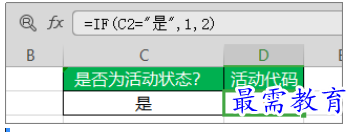• =IF(C2=”Yes”,1,2)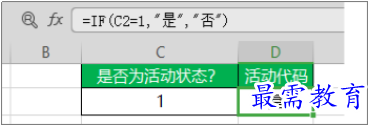• =IF(C2=1,”Yes”,”No”)

此外，还可将多个 IF 函数嵌套在一起来执行多个比较。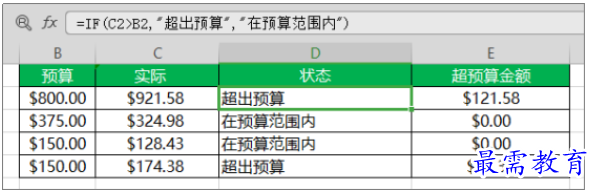• =IF(C2>B2,”Over Budget”,”Within Budget”)

（C2 大于 B2，则返回“Over Budget”，否则就返回“Within Budget”）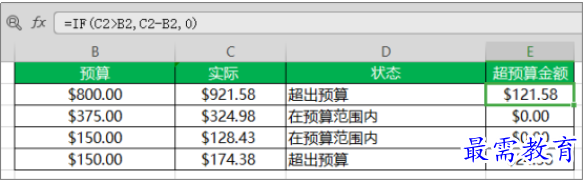• =IF(C2>B2,C2-B2,0)

E2 中的公式表示如果（实际值大于预算，则从实际金额中减去预算金额，否则就不返回任何结果）。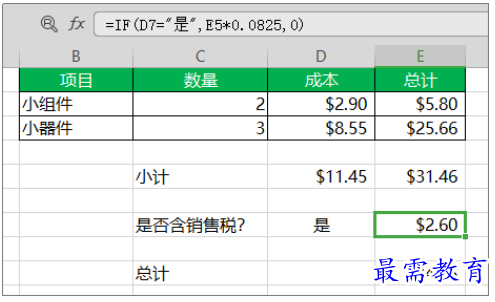• =IF(D7=”Yes”,E5*0.0825,0)，在此示例中，E7 中的公式表示如果：

（D7 = “Yes”，则计算 E5 * 8.25% 的总金额，否则就不应该付销售税，因此返回 0）

唯一的例外是使用 TRUE 和 FALSE 时，「表格（Excel）」能自动理解它们。

1、单元格中的 0（零）：value_if_true 或 value_if_False 参数无参数值。

2、#NAME? （显示在单元格中）：这通常意味着公式存在拼写错误。